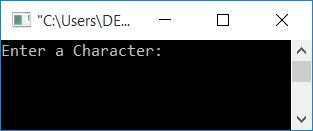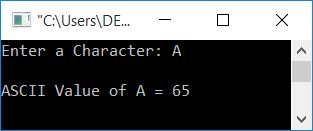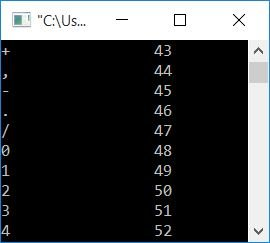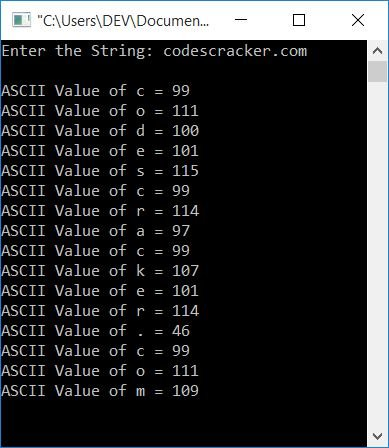# C Program to Print the ASCII Value of a Character

In this article, you will learn and get code for printing the ASCII value(s) of a character, all characters, or all characters in a string (given by the user at run-time). So here is the list of programs you will go through over here:

### What is ASCII?

ASCII stands for "American Standard Code for Information Interchange." It is a character encoding standard for electronic communication.

## Print a character's ASCII value in C

The question is: write a program in C that receives a character as input and prints its ASCII value as output. The answer to this question is:

```#include<stdio.h>
#include<conio.h>
int main()
{
char ch;
int i;
printf("Enter a Character: ");
scanf("%c", &ch);
i = ch;
printf("\nASCII Value of %c = %d", ch, i);
getch();
return 0;
}```

This program was built and run in the Code::Blocks IDE. Here is its sample run:Now supply any character, say A, and press the ENTER key to get its ASCII value:The statement,

`i = ch;`

initializes the ASCII value of a character (stored in the ch variable) to i, because i is of the int (inter) type. Therefore, the ASCII value of ch gets initialized to i. Print the value of i.

Note: You can also print the ASCII value directly without using the above statement. To do this, just use the format specifier %d to print the ASCII value and %c to print the character back on output.

## Print the ASCII value of all characters in C

The question is: write a program in C that prints the ASCII value of all characters. Here is its answer. In this program, without initializing the character as an integer type variable, we've just printed the value using the format specifier.

```#include<stdio.h>
#include<conio.h>
int main()
{
int i;
printf("Character \t ASCII Value\n\n");
for(i=0; i<255; i++)
printf("%c \t\t %d\n", i, i);
getch();
return 0;
}```

If you run the preceding program, you will see the following output:The above snapshot is just a small part of the output produced by the previous program. Because there are 254 characters printed, we are unable to display them all at once, so you have only seen a portion of the output.

## C: Print the ASCII value of all characters in a string

The question is: write a program in C that receives a string as input and prints the ASCII value of all the characters in the given string. Here is its answer:

```#include<stdio.h>
#include<conio.h>
int main()
{
char str;
int i=0;
printf("Enter the String: ");
gets(str);
while(str[i]!='\0')
{
printf("\nASCII Value of %c = %d", str[i], str[i]);
i++;
}
getch();
return 0;
}```

Assume that after running the preceding program, the user entered codescracker.com as a string input. Therefore, here is the output produced by the above program:#### The same program in different languages

C Quiz

« Previous Program Next Program »